# Estimate a GEV on the Fremantle sea-levels data¶

In this example, we illustrate various techniques of extreme value modeling applied to the annual maximum sea-levels recorded at Fremantle, near Perth, western Australia, over the period 1897-1989. Readers should refer to [coles2001] to get more details.

We illustrate techniques to:

• estimate a stationary and a non stationary GEV,

• estimate a return level,

using:

• the log-likelihood function,

• the profile log-likelihood function.

First, we load the Fremantle dataset of the annual maximum sea-levels. We start by looking at them through time. The data also contain the annual mean value of the Southern Oscillation Index (SOI), which is a proxy for meteorological volatility due to effects such as El Nino.

import openturns as ot
import openturns.viewer as otv
import openturns.experimental as otexp
from openturns.usecases import coles

data = coles.Coles().fremantle
print(data[:5])
graph = ot.Graph(
"Annual maximum sea-levels at Fremantle", "year", "level (m)", True, ""
)
cloud = ot.Cloud(data[:, :2])
cloud.setColor("red")
graph.setIntegerXTick(True)
view = otv.View(graph)[ Year     SeaLevel SOI      ]
0 : [ 1897        1.58    -0.67  ]
1 : [ 1898        1.71     0.57  ]
2 : [ 1899        1.4      0.16  ]
3 : [ 1900        1.34    -0.65  ]
4 : [ 1901        1.43     0.06  ]


We select the sea-levels column.

sample = data[:, 1]


Stationary GEV modeling via the log-likelihood function

We first assume that the dependence through time is negligible, so we first model the data as independent observations over the observation period. We estimate the parameters of the GEV distribution by maximizing the log-likelihood of the data.

factory = ot.GeneralizedExtremeValueFactory()
result_LL = factory.buildMethodOfLikelihoodMaximizationEstimator(sample)


We get the fitted GEV and its parameters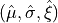.

fitted_GEV = result_LL.getDistribution()
desc = fitted_GEV.getParameterDescription()
param = fitted_GEV.getParameter()
print(", ".join([f"{p}: {value:.3f}" for p, value in zip(desc, param)]))

mu: 1.482, sigma: 0.141, xi: -0.217


We get the asymptotic distribution of the estimator. In that case, the asymptotic distribution is normal.

parameterEstimate = result_LL.getParameterDistribution()
print("Asymptotic distribution of the estimator : ")
print(parameterEstimate)

Asymptotic distribution of the estimator :
Normal(mu = [1.48231,0.141241,-0.217052], sigma = [0.0176728,0.0105976,0.0776361], R = [[  1         0.15748  -0.482101 ]
[  0.15748   1        -0.411378 ]
[ -0.482101 -0.411378  1        ]])


We get the covariance matrix and the standard deviation of.

print("Cov matrix = \n", parameterEstimate.getCovariance())
print("Standard dev = ", parameterEstimate.getStandardDeviation())

Cov matrix =
[[  0.000312329  2.94943e-05 -0.000661467 ]
[  2.94943e-05  0.000112309 -0.000338463 ]
[ -0.000661467 -0.000338463  0.00602736  ]]
Standard dev =  [0.0176728,0.0105976,0.0776361]


We get the marginal confidence intervals of order 0.95.

order = 0.95
for i in range(3):
ci = parameterEstimate.getMarginal(i).computeBilateralConfidenceInterval(order)
print(desc[i] + ":", ci)

mu: [1.44767, 1.51694]
sigma: [0.12047, 0.162012]
xi: [-0.369216, -0.0648881]


At last, we can validate the inference result thanks the 4 usual diagnostic plots:

• the probability-probability pot,

• the quantile-quantile pot,

• the return level plot,

• the empirical distribution function.

validation = otexp.GeneralizedExtremeValueValidation(result_LL, sample)
graph = validation.drawDiagnosticPlot()
view = otv.View(graph)Stationary GEV modeling via the profile log-likelihood function

Now, we use the profile log-likehood function rather than log-likehood function to estimate the parameters of the GEV.

result_PLL = factory.buildMethodOfProfileLikelihoodMaximizationEstimator(sample)


The following graph allows one to get the profile log-likelihood plot. It also indicates the optimal value of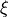, the maximum profile log-likelihood and the confidence interval forof order 0.95 (which is the default value).

order = 0.95
result_PLL.setConfidenceLevel(order)
view = otv.View(result_PLL.drawProfileLikelihoodFunction())We can get the numerical values of the confidence interval: it appears to be a bit smaller than the interval obtained with the log-likelihood function. Note that if the order requested is too high, the confidence interval might not be calculated because one of its bound is out of the definition domain of the log-likelihood function.

try:
print("Confidence interval for xi = ", result_PLL.getParameterConfidenceInterval())
except Exception as ex:
print(type(ex))
pass

Confidence interval for xi =  [-0.334109, -0.0802265]


Return level estimate from the estimated stationary GEV

We estimate the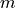-block return level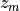: it is computed as a particular quantile of the GEV model estimated using the log-likelihood function. We just have to use the maximum log-likelihood estimator built in the previous section.

As the data are annual sea-levels, each block corresponds to one year: the 10-year return level corresponds to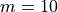and the 100-year return level corresponds to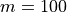.

The method provides the asymptotic distribution of the estimator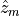which mean is the return-level estimate.

zm_10 = factory.buildReturnLevelEstimator(result_LL, 10.0)
return_level_10 = zm_10.getMean()
print("Maximum log-likelihood function : ")
print(f"10-year return level = {return_level_10}")
return_level_ci10 = zm_10.computeBilateralConfidenceInterval(0.95)
print(f"CI = {return_level_ci10}")

Maximum log-likelihood function :
10-year return level = [1.73376]
CI = [1.68892, 1.7786]

zm_100 = factory.buildReturnLevelEstimator(result_LL, 100.0)
return_level_100 = zm_100.getMean()
print(f"100-year return level = {return_level_100}")
return_level_ci100 = zm_100.computeBilateralConfidenceInterval(0.95)
print(f"CI = {return_level_ci100}")

100-year return level = [1.89328]
CI = [1.79336, 1.99319]


Return level estimate via the profile log-likelihood function of a stationary GEV

We can estimate the-block return leveldirectly from the data using the profile likelihood with respect to.

result_zm_10_PLL = factory.buildReturnLevelProfileLikelihoodEstimator(sample, 10.0)
zm_10_PLL = result_zm_10_PLL.getParameter()
print(f"10-year return level (profile) = {zm_10_PLL}")

10-year return level (profile) = 1.7337304564424918


We can get the confidence interval of: once more, it appears to be a bit smaller than the interval obtained from the log-likelihood function. As for the confidence interval of, depending on the order requested, the interval might not be calculated.

result_zm_10_PLL.setConfidenceLevel(0.95)
try:
return_level_ci10 = result_zm_10_PLL.getParameterConfidenceInterval()
except Exception as ex:
print(type(ex))
pass
print("Maximum profile log-likelihood function : ")
print(f"CI={return_level_ci10}")

Maximum profile log-likelihood function :
CI=[1.69343, 1.78619]


We can also plot the profile log-likelihood function and get the confidence interval, the optimal value ofand its confidence interval.

view = otv.View(result_zm_10_PLL.drawProfileLikelihoodFunction())Non stationary GEV modeling via the log-likelihood function

If we look at the data carefully, we see that the pattern of variation has not remained constant over the observation period. There is an increase in the data through time. We want to model this dependence because a slight increase in extreme sea-levels might have a significant impact on the safety of coastal flood defenses.

We have define the functional basis for each parameter of the GEV model. Even if we have the possibility to affect a time-varying model to each of the 3 parameters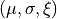, it is strongly recommended not to vary the parameterand to let it constant.

For numerical reasons, it is strongly recommended to normalize all the data as follows: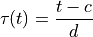where:

• the CenterReduce method where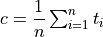is the mean time stamps and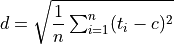is the standard deviation of the time stamps;

• the MinMax method where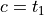is the initial time and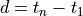the final time;

• the None method where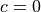and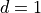: in that case, data are not normalized.

We suppose that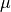is linear in time, and that the other parameters remain constant: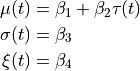constant = ot.SymbolicFunction(["t"], ["1.0"])
basis_lin = ot.Basis([constant, ot.SymbolicFunction(["t"], ["t"])])
basis_cst = ot.Basis([constant])
# basis for mu, sigma, xi
basis_coll = [basis_lin, basis_cst, basis_cst]

timeStamps = data[:, 0]


We can now estimate the list of coefficients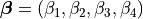using the log-likelihood of the data.

We test the 3 normalizing methods and both initial points in order to evaluate their impact on the results. We can see that:

• both normalization methods lead to the same result for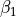,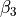and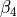(note that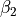depends on the normalization function),

• both initial points lead to the same result when the data have been normalized,

• it is very important to normalize all the data: if not, the result strongly depends on the initial point and it differs from the result obtained with normalized data. The results are not optimal in that case since the associated log-likelihood are much smaller than those obtained with normalized data.

initiPoint_list = list()
initiPoint_list.append("Gumbel")
initiPoint_list.append("Static")
normMethod_list = list()
normMethod_list.append("MinMax")
normMethod_list.append("CenterReduce")
normMethod_list.append("None")

print("Linear mu(t) model: ")
for normMeth in normMethod_list:
for initPoint in initiPoint_list:
print("normMeth, initPoint = ", normMeth, initPoint)
# The ot.Function() is the identity function.
result = factory.buildTimeVarying(
sample, timeStamps, basis_coll, ot.Function(), initPoint, normMeth
)
beta = result.getOptimalParameter()
print("beta1, beta2, beta3, beta4 = ", beta)
print("Max log-likelihood =  ", result.getLogLikelihood())

Linear mu(t) model:
normMeth, initPoint =  MinMax Gumbel
beta1, beta2, beta3, beta4 =  [1.38216,0.187033,0.124317,-0.125086]
Max log-likelihood =   49.912808020251134
normMeth, initPoint =  MinMax Static
beta1, beta2, beta3, beta4 =  [1.38227,0.186899,0.124343,-0.125475]
Max log-likelihood =   49.91281020707175
normMeth, initPoint =  CenterReduce Gumbel
beta1, beta2, beta3, beta4 =  [1.48016,0.0541499,0.124307,-0.124893]
Max log-likelihood =   49.91279553702213
normMeth, initPoint =  CenterReduce Static
beta1, beta2, beta3, beta4 =  [1.48024,0.0541138,0.124349,-0.125787]
Max log-likelihood =   49.91278966404141
normMeth, initPoint =  None Gumbel
beta1, beta2, beta3, beta4 =  [1.47155,1.67803e-05,0.211226,0.0876902]
Max log-likelihood =   26.490076768443522
normMeth, initPoint =  None Static
beta1, beta2, beta3, beta4 =  [1.4823,1.34614e-09,0.141241,-0.217051]
Max log-likelihood =   43.566619143025775


According to the previous results, we choose the MinMax normalization method and the Gumbel initial point. This initial point is cheaper than the Static one as it requires no optimization computation.

result_NonStatLL = factory.buildTimeVarying(
sample, timeStamps, basis_coll, ot.Function(), "Gumbel", "MinMax"
)
beta = result_NonStatLL.getOptimalParameter()
print("beta1, beta2, beta3, beta_4 = ", beta)
print(f"mu(t) = {beta:.4f} + {beta:.4f} * tau")
print(f"sigma = {beta:.4f}")
print(f"xi = {beta:.4f}")

beta1, beta2, beta3, beta_4 =  [1.38216,0.187033,0.124317,-0.125086]
mu(t) = 1.3822 + 0.1870 * tau
sigma = 0.1243
xi = -0.1251


You can get the expression of the normalizing function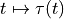:

normFunc = result_NonStatLL.getNormalizationFunction()
print("Function tau(t): ", normFunc)
print("c = ", normFunc.getEvaluation().getImplementation().getCenter())
print("1/d = ", normFunc.getEvaluation().getImplementation().getLinear()[0, 0])

Function tau(t):  class=LinearFunction name=Unnamed implementation=class=LinearEvaluation name=Unnamed center= constant= linear=[[ 0.0108696 ]]
c =  1897.0
1/d =  0.010869565217391304


You can get the function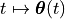where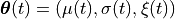.

functionTheta = result_NonStatLL.getParameterFunction()


We get the asymptotic distribution of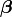to compute some confidence intervals of the estimates, for example of order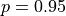.

dist_beta = result_NonStatLL.getParameterDistribution()
condifence_level = 0.95
for i in range(beta.getSize()):
lower_bound = dist_beta.getMarginal(i).computeQuantile((1 - condifence_level) / 2)[
0
]
upper_bound = dist_beta.getMarginal(i).computeQuantile((1 + condifence_level) / 2)[
0
]
print(
"Conf interval for beta_"
+ str(i + 1)
+ " = ["
+ str(lower_bound)
+ "; "
+ str(upper_bound)
+ "]"
)

Conf interval for beta_1 = [1.3261592601754428; 1.4381658634148222]
Conf interval for beta_2 = [0.09344563045761152; 0.2806199116219121]
Conf interval for beta_3 = [0.10189124975098564; 0.14674373833543314]
Conf interval for beta_4 = [-0.29294234394146657; 0.0427699012822019]


In order to compare different modelings, we get the optimal log-likelihood of the data for both stationary and non stationary models. The difference is significant enough to be in favor of the non stationary model.

print("Max log-likelihood: ")
print("Stationary model =  ", result_LL.getLogLikelihood())
print("Non stationary linear mu(t) model =  ", result_NonStatLL.getLogLikelihood())

Max log-likelihood:
Stationary model =   43.566611777651026
Non stationary linear mu(t) model =   49.912808020251134


In order to draw some diagnostic plots similar to those drawn in the stationary case, we refer to the following result: if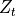is a non stationary GEV model parametrized by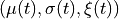, then the standardized variables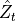defined by: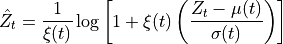have the standard Gumbel distribution which is the GEV model with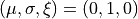.

As a result, we can validate the inference result thanks the 4 usual diagnostic plots:

• the probability-probability pot,

• the quantile-quantile pot,

• the return level plot,

• the data histogram and the desnity of the fitted model.

using the transformed data compared to the Gumbel model. We can see that the adequation is better than with the stationary model.

graph = result_NonStatLL.drawDiagnosticPlot()
view = otv.View(graph)We can draw the mean function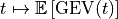. Be careful, it is not the function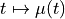. As a matter of fact, the mean is defined for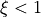only and in that case, for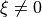, we have: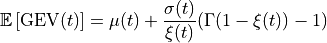and for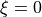, we have: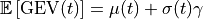where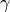is the Euler constant.

We can also draw the function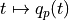where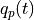is the quantile of order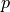of the GEV distribution at time. Here,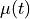is a linear function and the other parameters are constant, so the mean and the quantile functions are also linear functions.

graph = ot.Graph(
r"Annual maximum sea-levels at Fremantle - Linear $\mu(t)$",
"year",
"level (m)",
True,
"",
)
graph.setIntegerXTick(True)
# data
cloud = ot.Cloud(data[:, :2])
cloud.setColor("red")
# mean function
meandata = [
result_NonStatLL.getDistribution(t).getMean() for t in data[:, 0].asPoint()
]
curve_meanPoints = ot.Curve(data[:, 0].asPoint(), meandata)
# quantile function
graphQuantile = result_NonStatLL.drawQuantileFunction(0.95)
drawQuant = graphQuantile.getDrawable(0)
drawQuant = graphQuantile.getDrawable(0)
drawQuant.setLineStyle("dashed")
graph.setLegends(["data", "mean function", "quantile 0.95  function"])
graph.setLegendPosition("bottomright")
view = otv.View(graph)At last, we can test the validity of the stationary model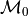relative to the model with time varying parameters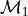. The modelis parametrized by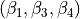and the modelis parametrized by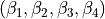: so we have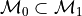.

We use the Likelihood Ratio test. The null hypothesis is the stationary model. The Type I error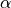is taken equal to 0.05.

This test confirms that the dependence through time is not negligible: it means that the linearcomponent explains a large variation in the data.

llh_LL = result_LL.getLogLikelihood()
llh_NonStatLL = result_NonStatLL.getLogLikelihood()
modelM0_Nb_param = 3
modelM1_Nb_param = 4
resultLikRatioTest = ot.HypothesisTest.LikelihoodRatioTest(
modelM0_Nb_param, llh_LL, modelM1_Nb_param, llh_NonStatLL, 0.05
)
accepted = resultLikRatioTest.getBinaryQualityMeasure()
print(
f"Hypothesis H0 (stationary model) vs H1 (linear mu(t) model):  accepted ? = {accepted}"
)

Hypothesis H0 (stationary model) vs H1 (linear mu(t) model):  accepted ? = False


We detail the statistics of the Likelihood Ratio test: the deviance statistics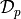follows a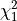distribution. The modelis rejected if the deviance statistics estimated on the data is greater than the threshold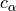or if the p-value is less than the Type I error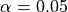.

print(f"Dp={resultLikRatioTest.getStatistic():.2f}")
print(f"alpha={resultLikRatioTest.getThreshold():.2f}")
print(f"p-value={resultLikRatioTest.getPValue():.2f}")

Dp=12.69
alpha=0.05
p-value=0.00


We can perform the same study with a quadratic model foror a linear model forand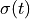: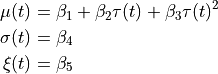or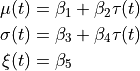For each model, we give the log-likelihood values and we test the validity of each model with respect to the non stationary model whereis linear. We notice that there is no evidence to adopt a quadratic model fornor a linear model forand: the optimal log-likelihood for each model is very near the likelihood we obtained with a linear model foronly. It means that these both models do not bring significant improvements with respect to model tested before.

basis_quad = ot.Basis(
[constant, ot.SymbolicFunction(["t"], ["t"]), ot.SymbolicFunction(["t"], ["t^2"])]
)
basis_coll_3 = [basis_lin, basis_lin, basis_cst]
result_NonStatLL_2 = factory.buildTimeVarying(
sample, timeStamps, basis_coll_2, ot.Function(), "Gumbel", "MinMax"
)
result_NonStatLL_3 = factory.buildTimeVarying(
sample, timeStamps, basis_coll_3, ot.Function(), "Gumbel", "MinMax"
)
print("Max log-likelihood = ")
print("Non stationary quadratic mu(t) model = ", result_NonStatLL_2.getLogLikelihood())
print(
"Non stationary linear mu(t) and sigma(t) model = ",
result_NonStatLL_3.getLogLikelihood(),
)
llh_LL = result_LL.getLogLikelihood()
llh_NonStatLL_2 = result_NonStatLL_2.getLogLikelihood()
llh_NonStatLL_3 = result_NonStatLL_3.getLogLikelihood()
resultLikRatioTest_2 = ot.HypothesisTest.LikelihoodRatioTest(
4, llh_NonStatLL, 5, llh_NonStatLL_2, 0.05
)
resultLikRatioTest_3 = ot.HypothesisTest.LikelihoodRatioTest(
4, llh_NonStatLL, 5, llh_NonStatLL_3, 0.05
)
accepted_2 = resultLikRatioTest_2.getBinaryQualityMeasure()
accepted_3 = resultLikRatioTest_3.getBinaryQualityMeasure()
print(
f"Hypothesis H0 (linear mu(t) model) vs H1 (quadratic mu(t) model):  accepted ? = {accepted_2}"
)
print(
f"Hypothesis H0 (linear mu(t) model) vs H1 (linear mu(t) and sigma(t) model):  accepted ? = {accepted_3}"
)

Max log-likelihood =
Non stationary quadratic mu(t) model =  50.65441648784694
Non stationary linear mu(t) and sigma(t) model =  50.70305201922592
Hypothesis H0 (linear mu(t) model) vs H1 (quadratic mu(t) model):  accepted ? = True
Hypothesis H0 (linear mu(t) model) vs H1 (linear mu(t) and sigma(t) model):  accepted ? = True

otv.View.ShowAll()


Total running time of the script: ( 0 minutes 5.702 seconds)